# Identify and model points lines and planes Identify

• Slides: 24• Identify and model points, lines, and planes. • Identify intersecting lines and planes.Content Standards G. CO. 1 Know precise definitions of angle, circle, perpendicular line, parallel line, and line segment, based on the undefined notions of point, line, distance along a line, and distance around a circular arc. Mathematical Practices 4 Model with mathematics. 6 Attend to precision.• undefined term • definition • point • defined term • line • space • plane • collinear • coplanar • intersection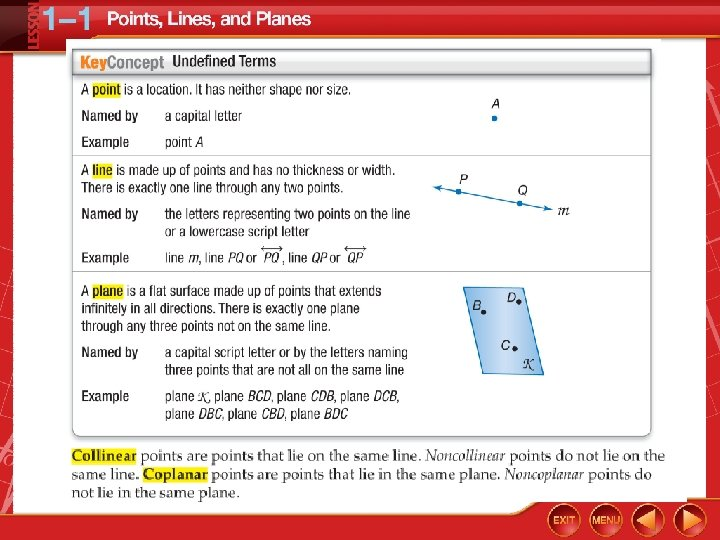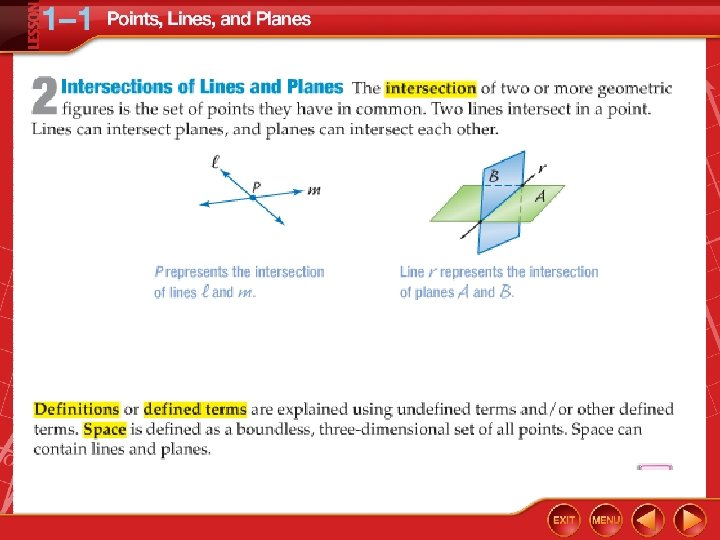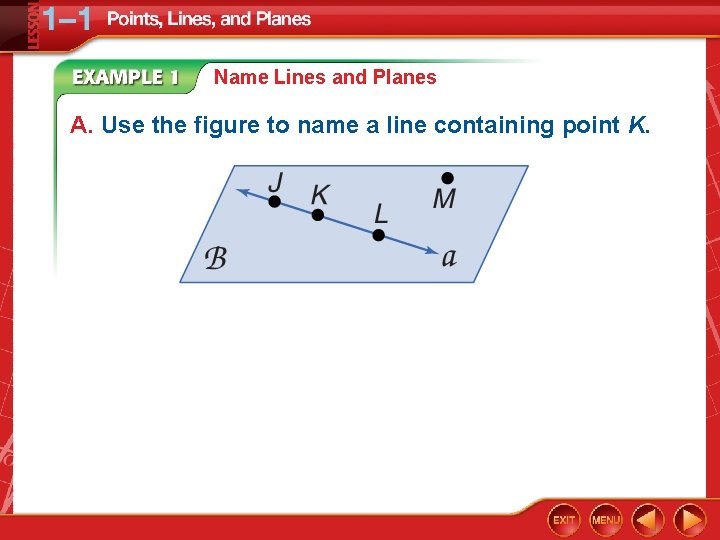Name Lines and Planes A. Use the figure to name a line containing point K.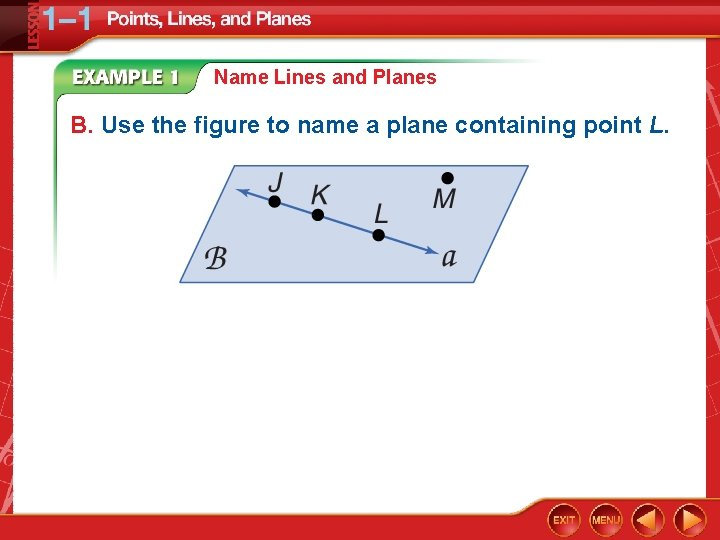Name Lines and Planes B. Use the figure to name a plane containing point L.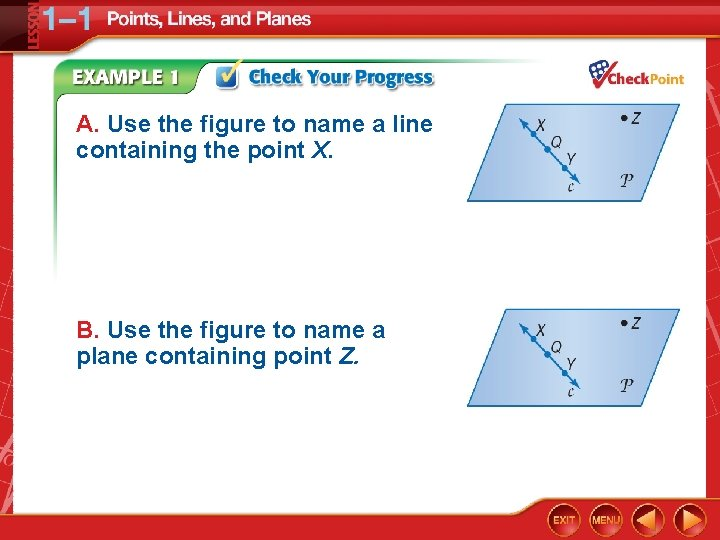A. Use the figure to name a line containing the point X. B. Use the figure to name a plane containing point Z.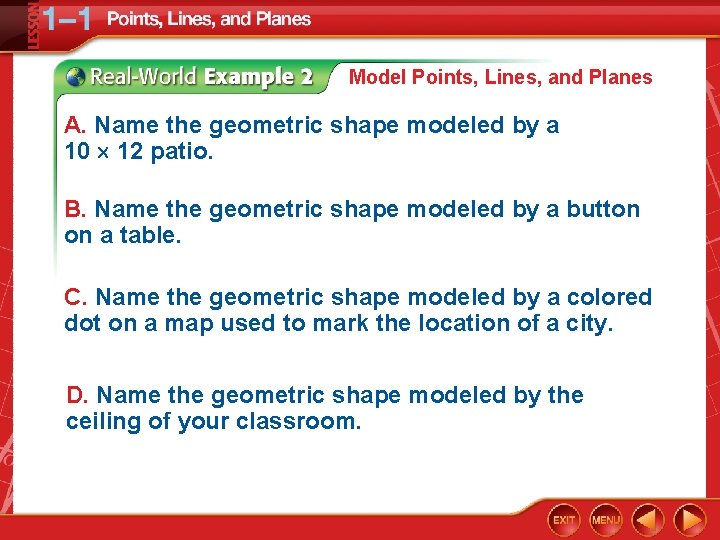Model Points, Lines, and Planes A. Name the geometric shape modeled by a 10 12 patio. B. Name the geometric shape modeled by a button on a table. C. Name the geometric shape modeled by a colored dot on a map used to mark the location of a city. D. Name the geometric shape modeled by the ceiling of your classroom.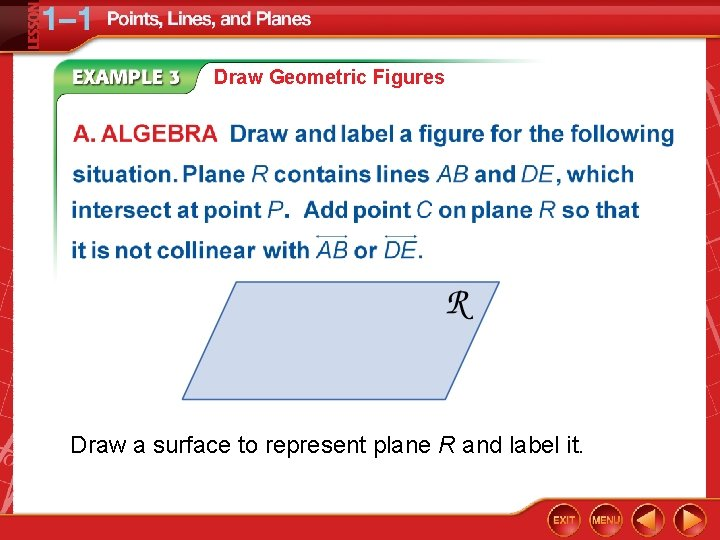Draw Geometric Figures Draw a surface to represent plane R and label it.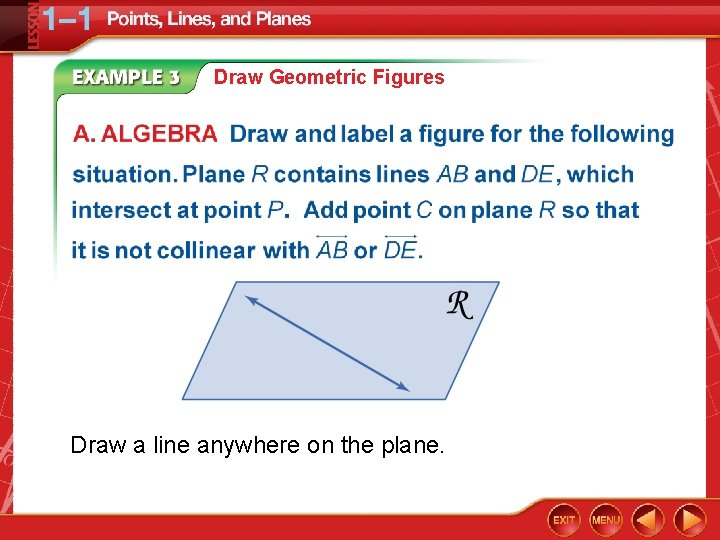Draw Geometric Figures Draw a line anywhere on the plane.Draw Geometric Figures Draw dots on the line for point A and B. Label the points.Draw Geometric Figures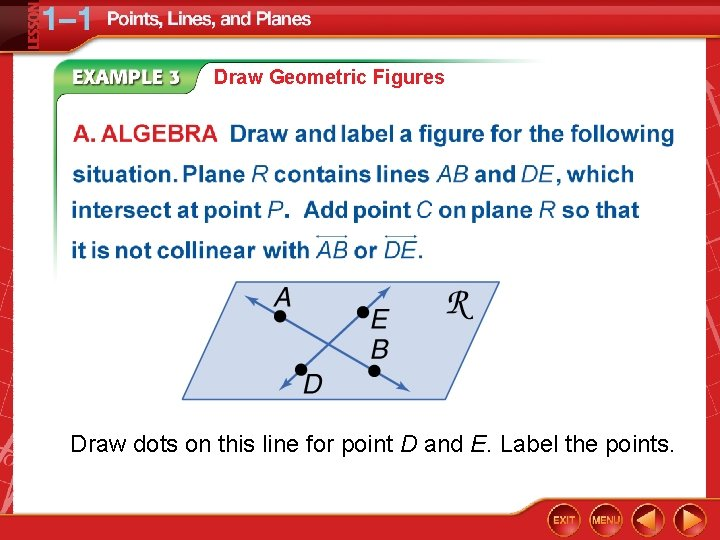Draw Geometric Figures Draw dots on this line for point D and E. Label the points.Draw Geometric Figures Label the intersection point of the two lines as P.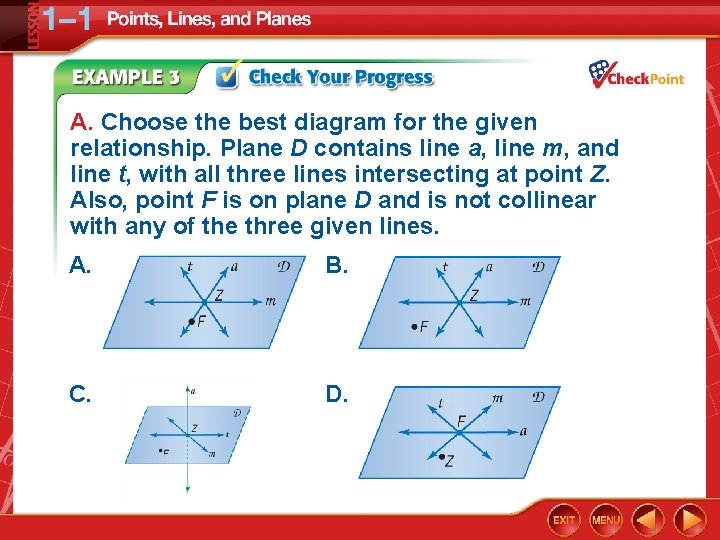A. Choose the best diagram for the given relationship. Plane D contains line a, line m, and line t, with all three lines intersecting at point Z. Also, point F is on plane D and is not collinear with any of the three given lines. A. B. C. D.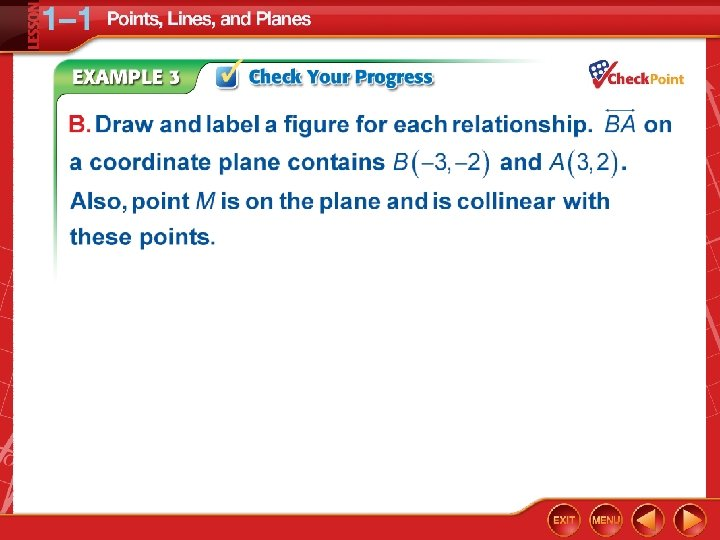Interpret Drawings A. How many planes appear in this figure?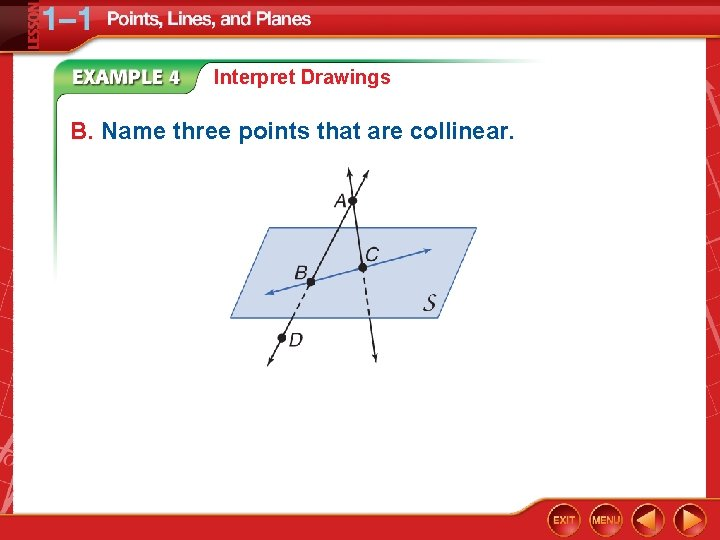Interpret Drawings B. Name three points that are collinear.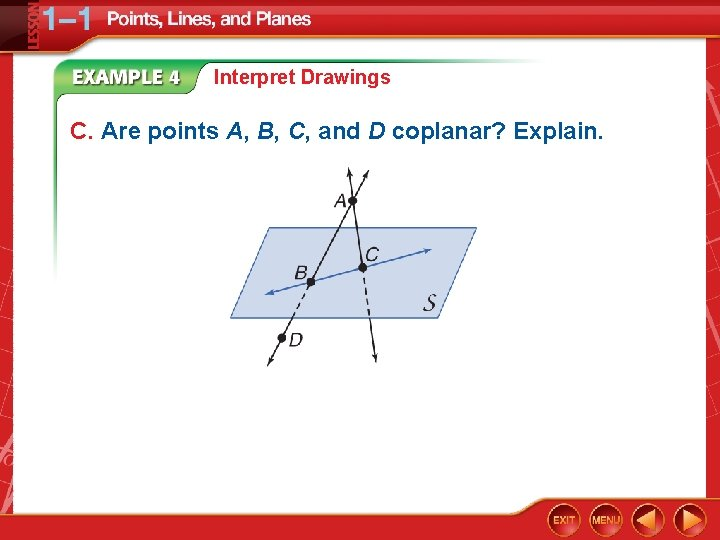Interpret Drawings C. Are points A, B, C, and D coplanar? Explain.Interpret Drawings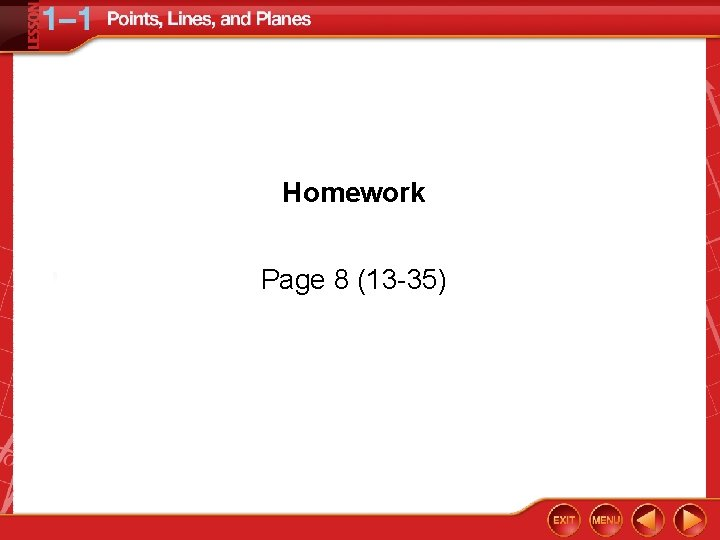Homework Page 8 (13 -35)